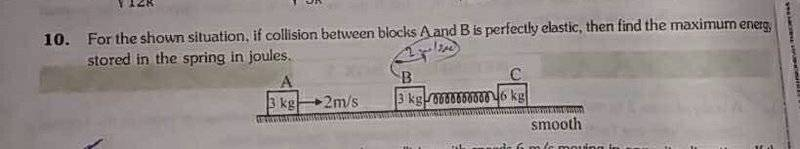# Energy stored in a spring

## Homework Statement## The Attempt at a Solution

If I work in the lab frame I get the correct answer 4J .

But I do not understand why I do not get the correct answer if I work in the moving frame of block B .

After collision with block A , block B starts moving with 2m/s towards right .

Now in the frame of B , initial velocity of C is 2m/s towards left . Total initial mechanical energy = (1/2)(6)(22) = 12J .

In this frame spring will have maximum compression when C is at rest .That means maximum potential energy should be 12 J .

What is the mistake ?

The same problem arises if I work in the frame of block C .

So why is it that energy conservation is not holding true in the moving frames ?

#### Attachments

Last edited:

•